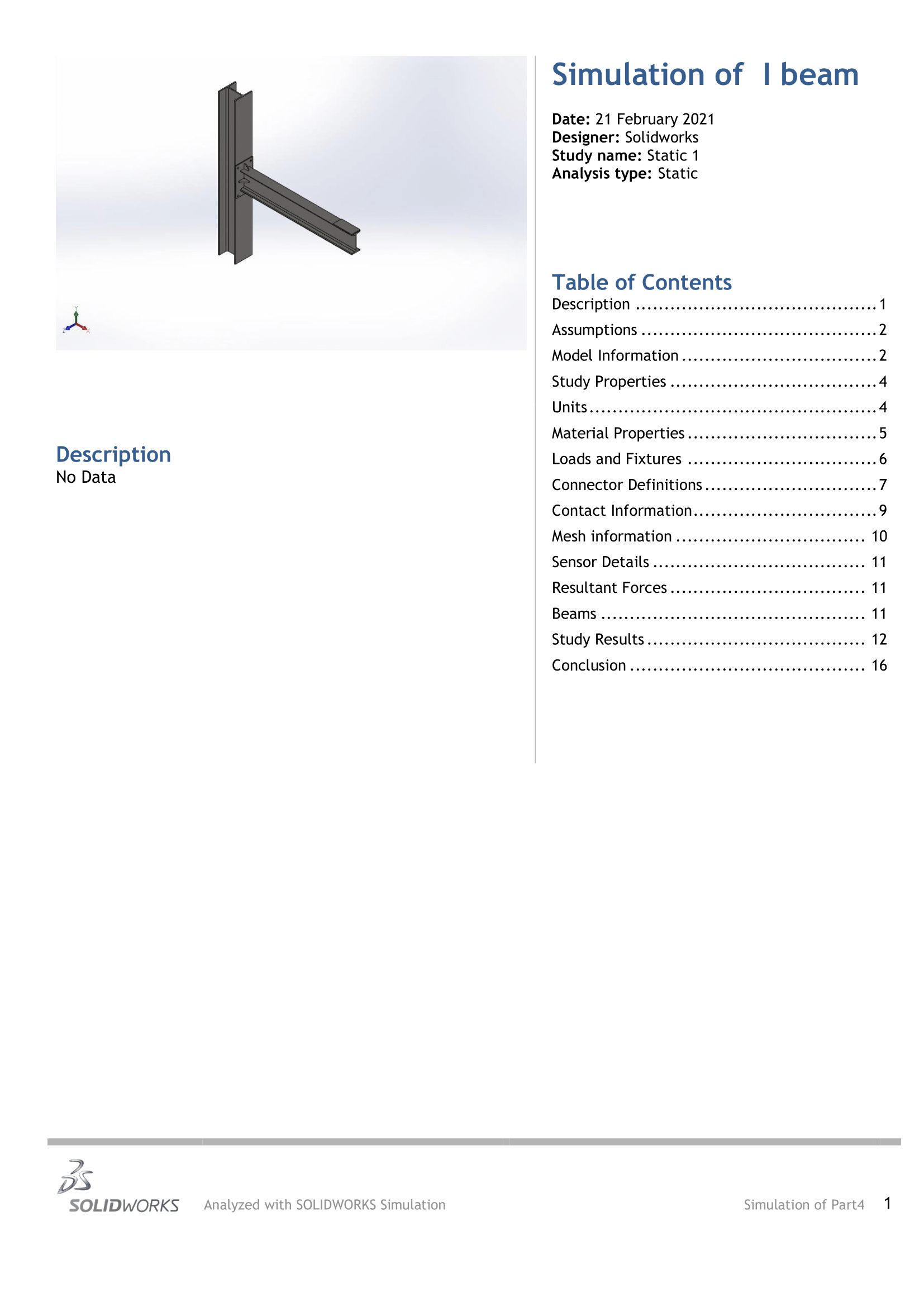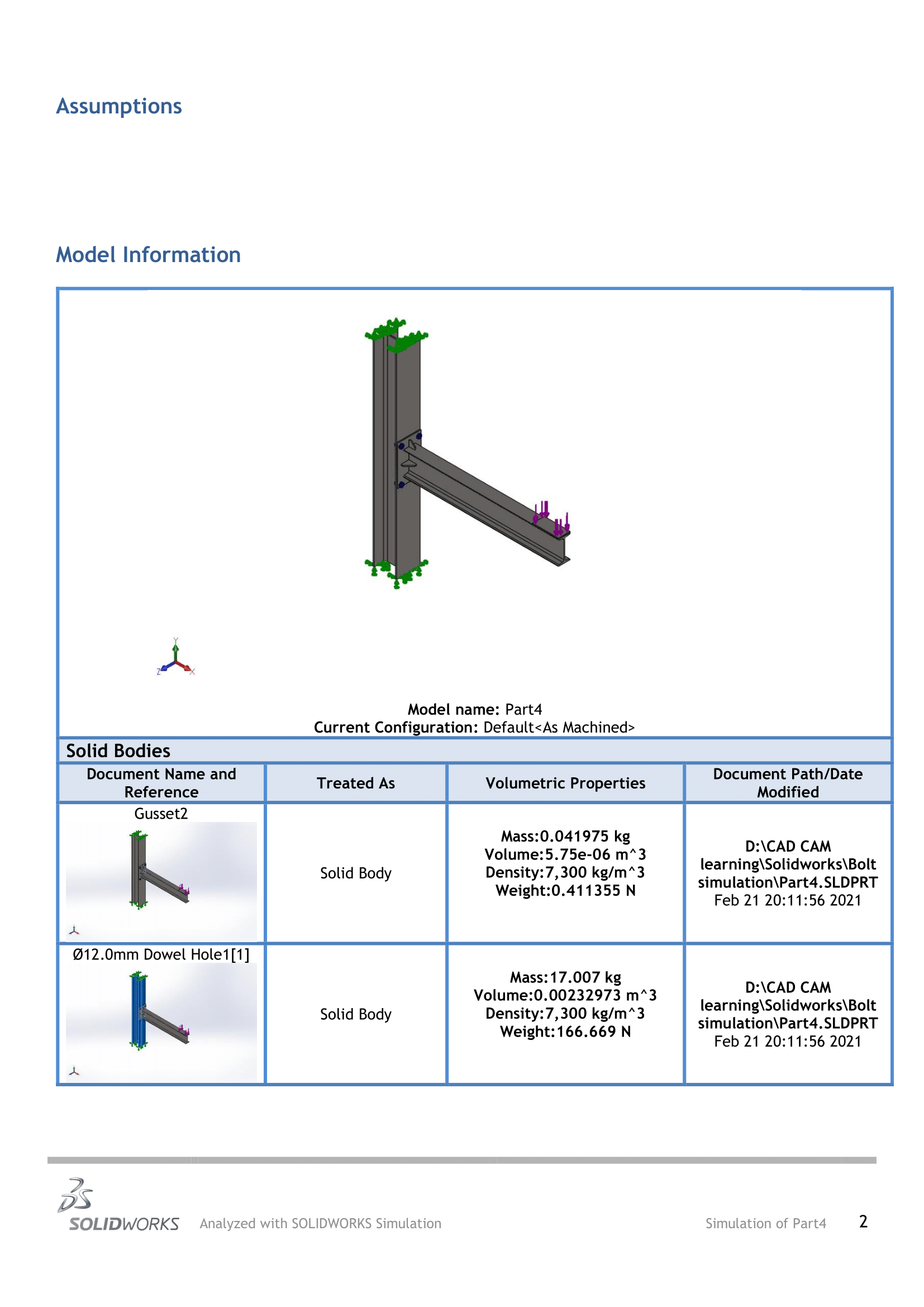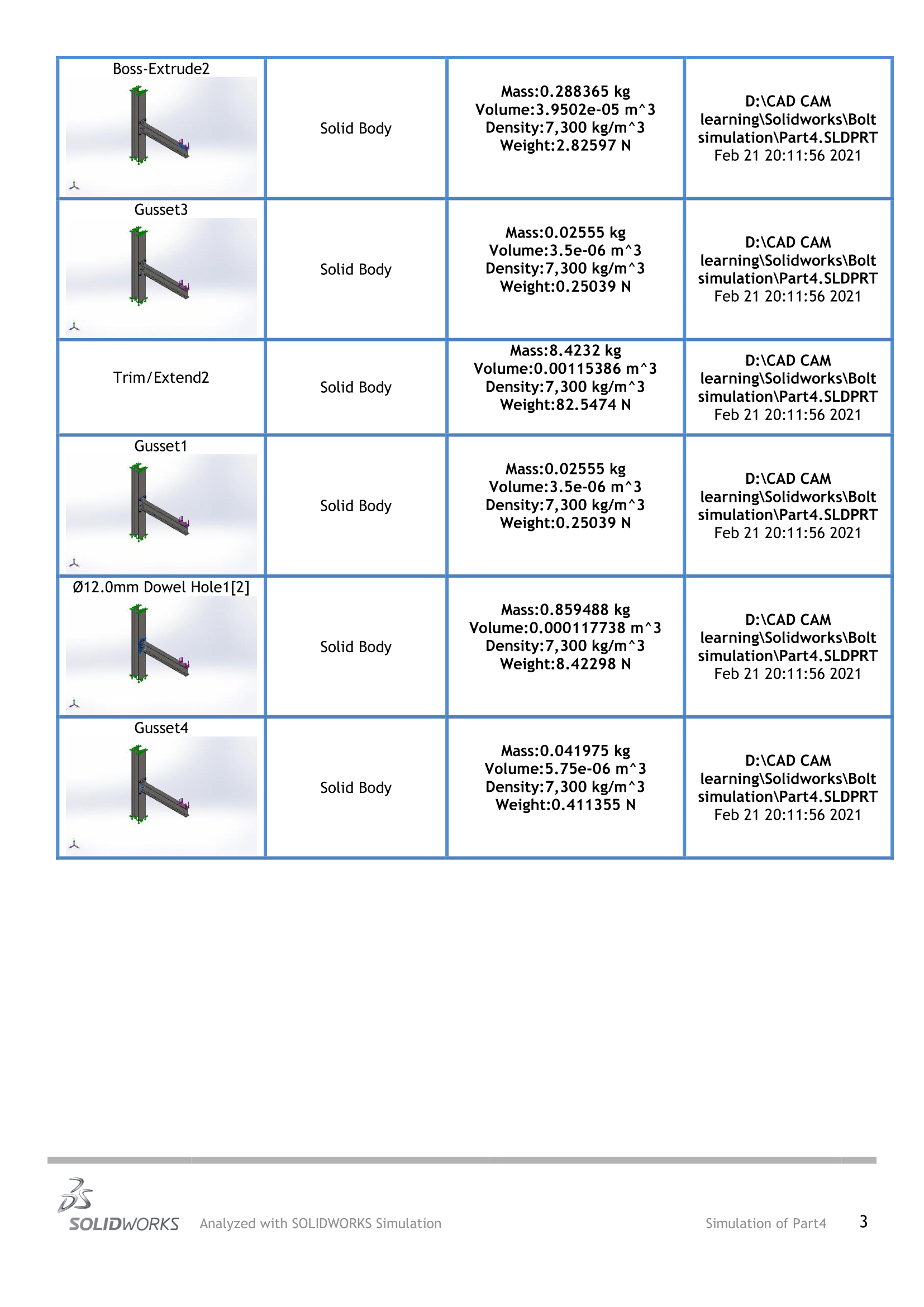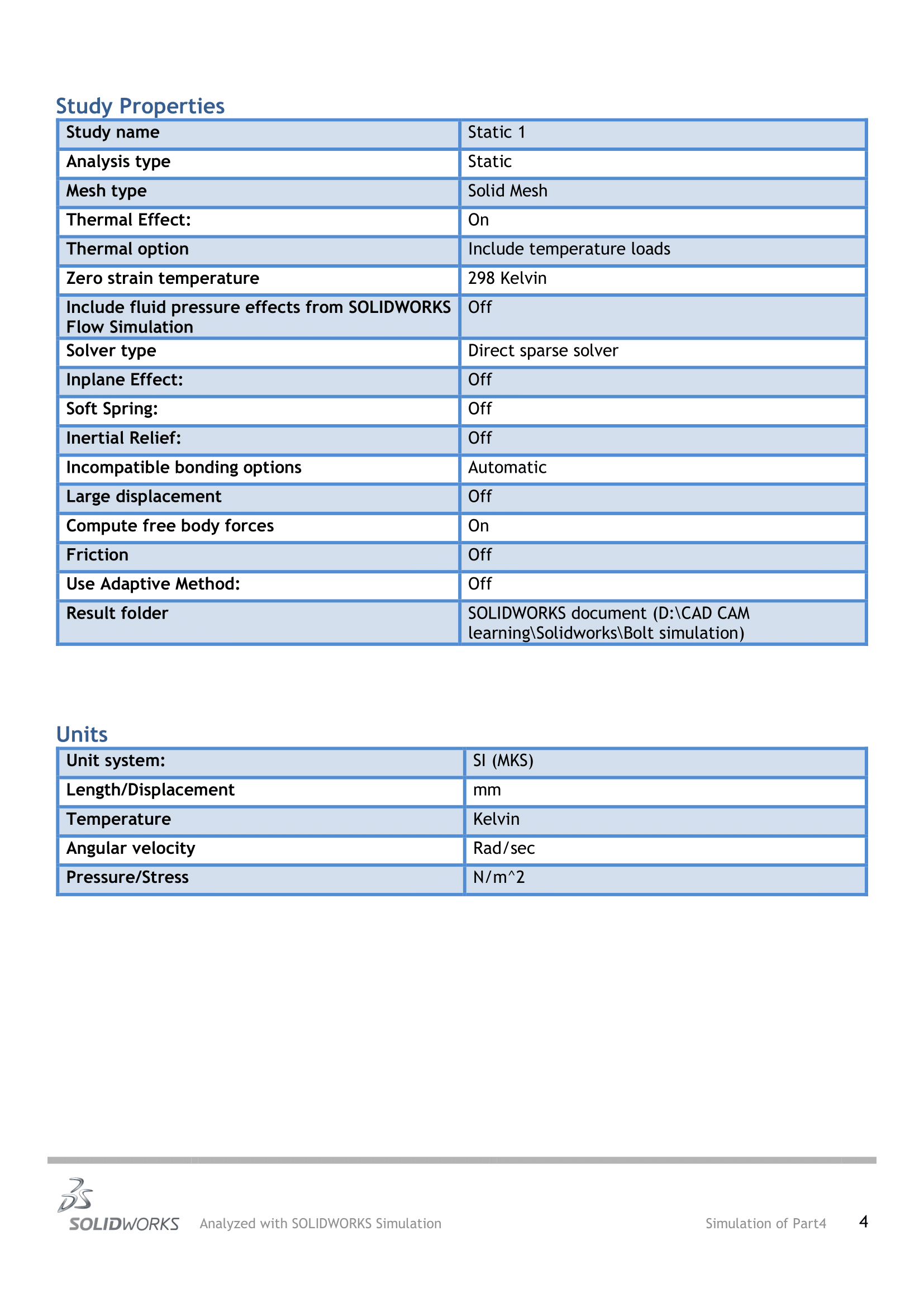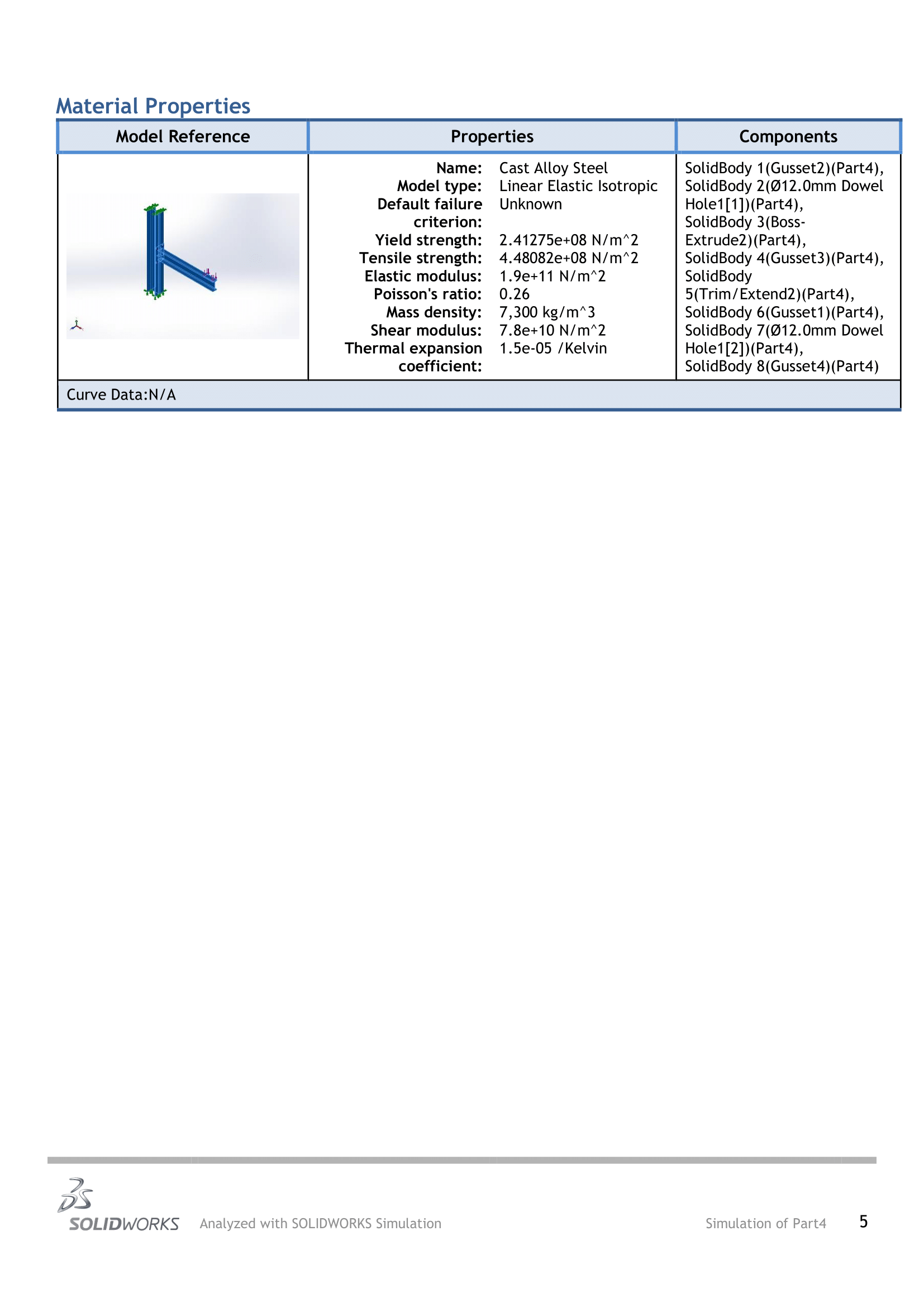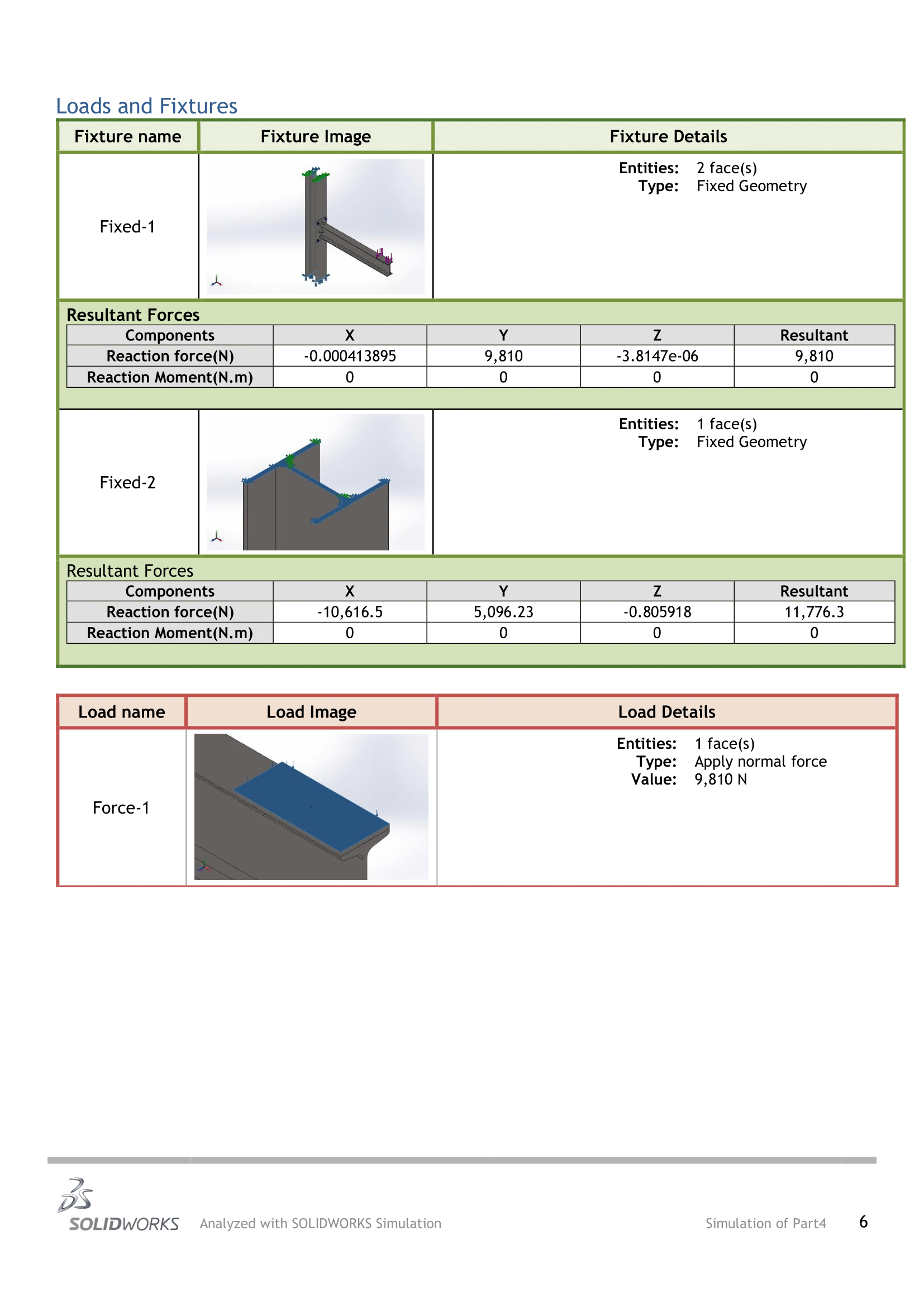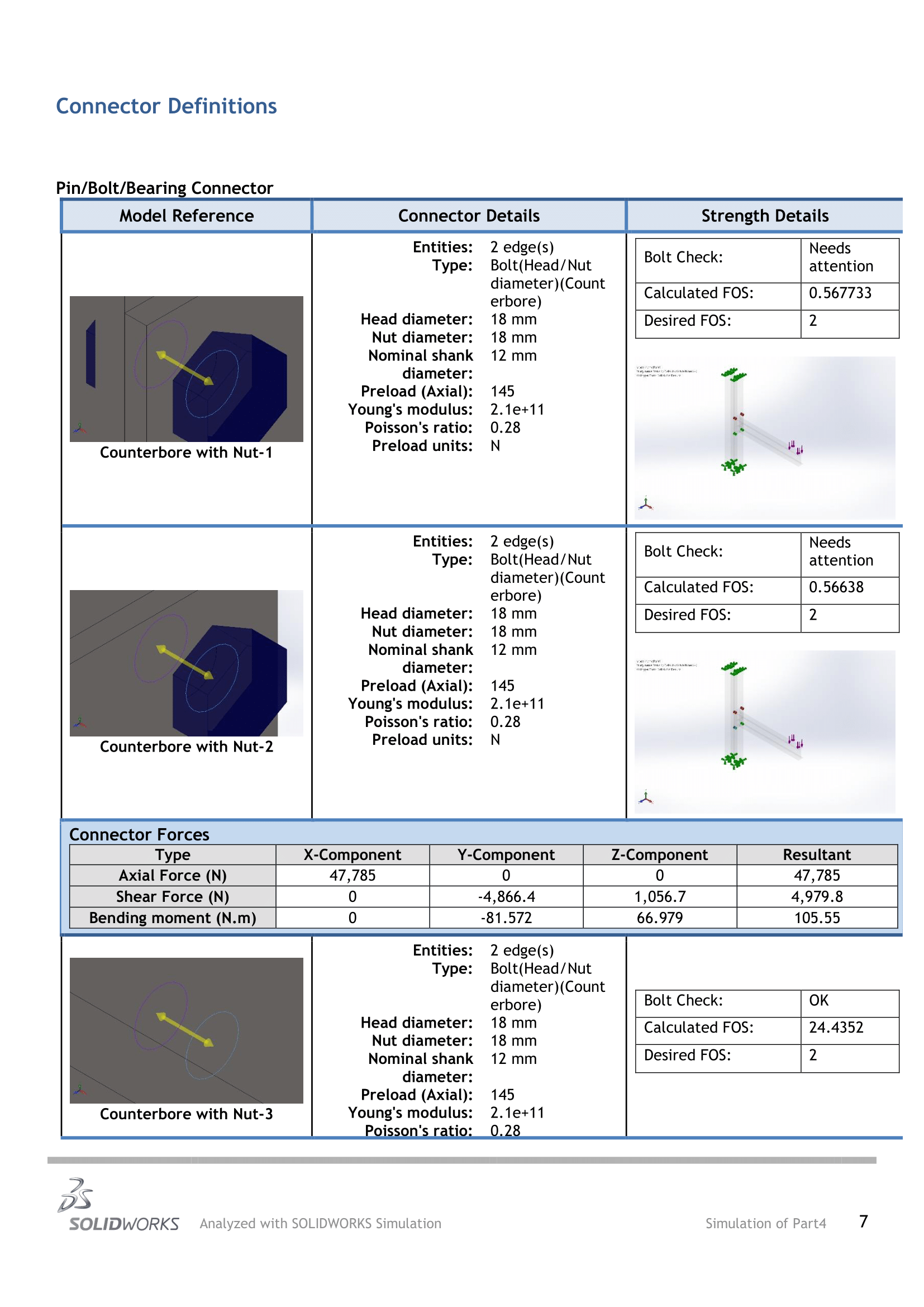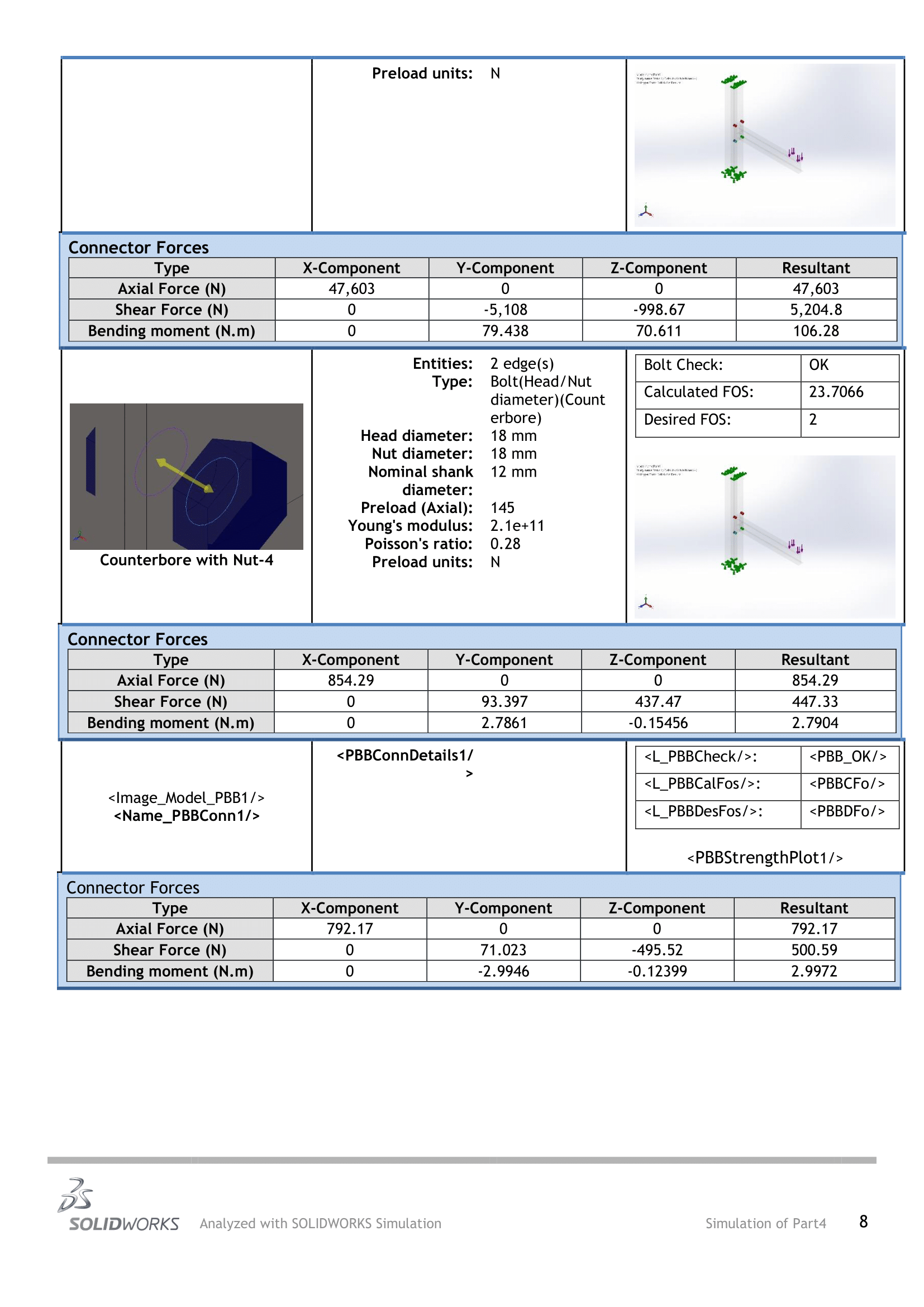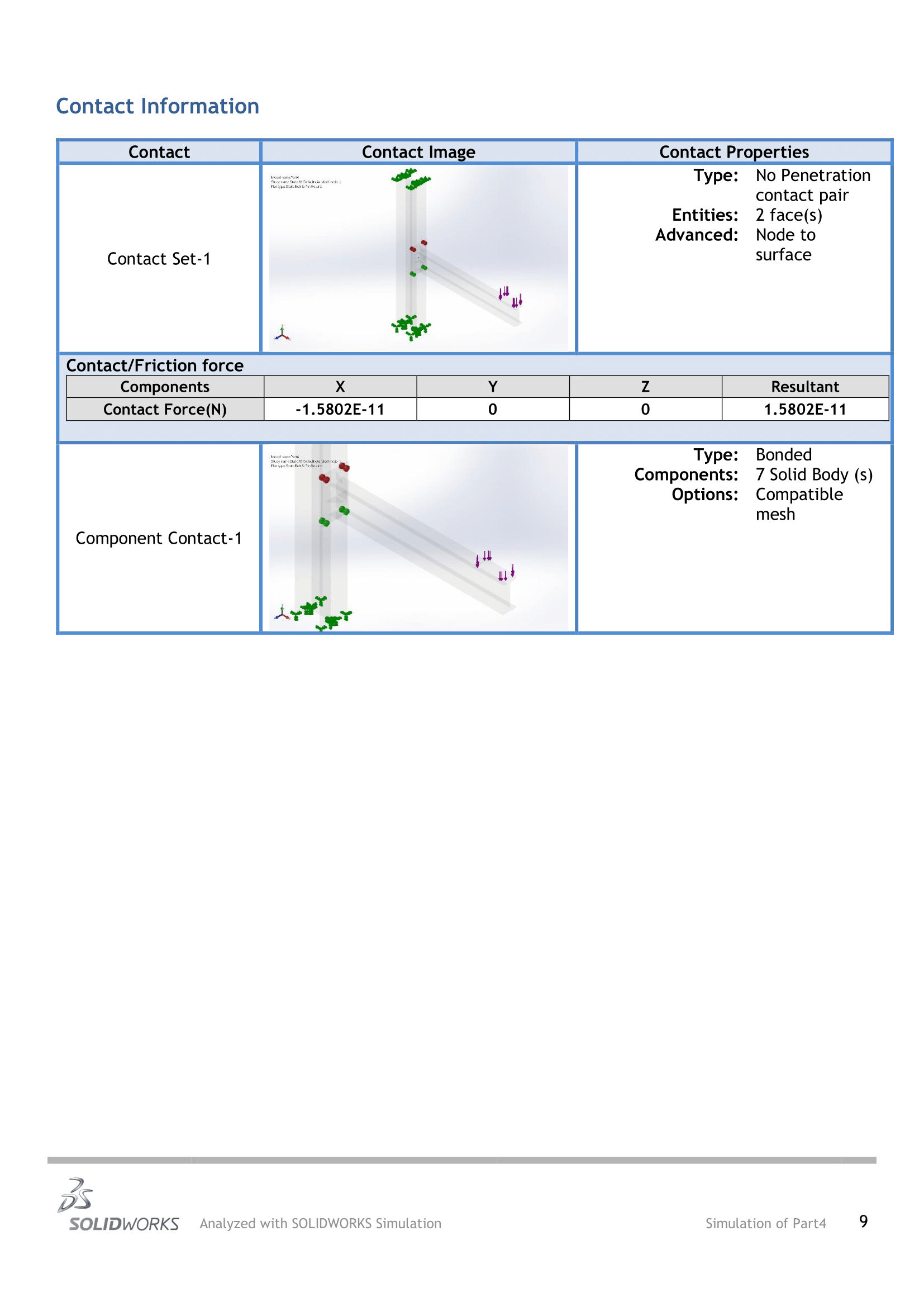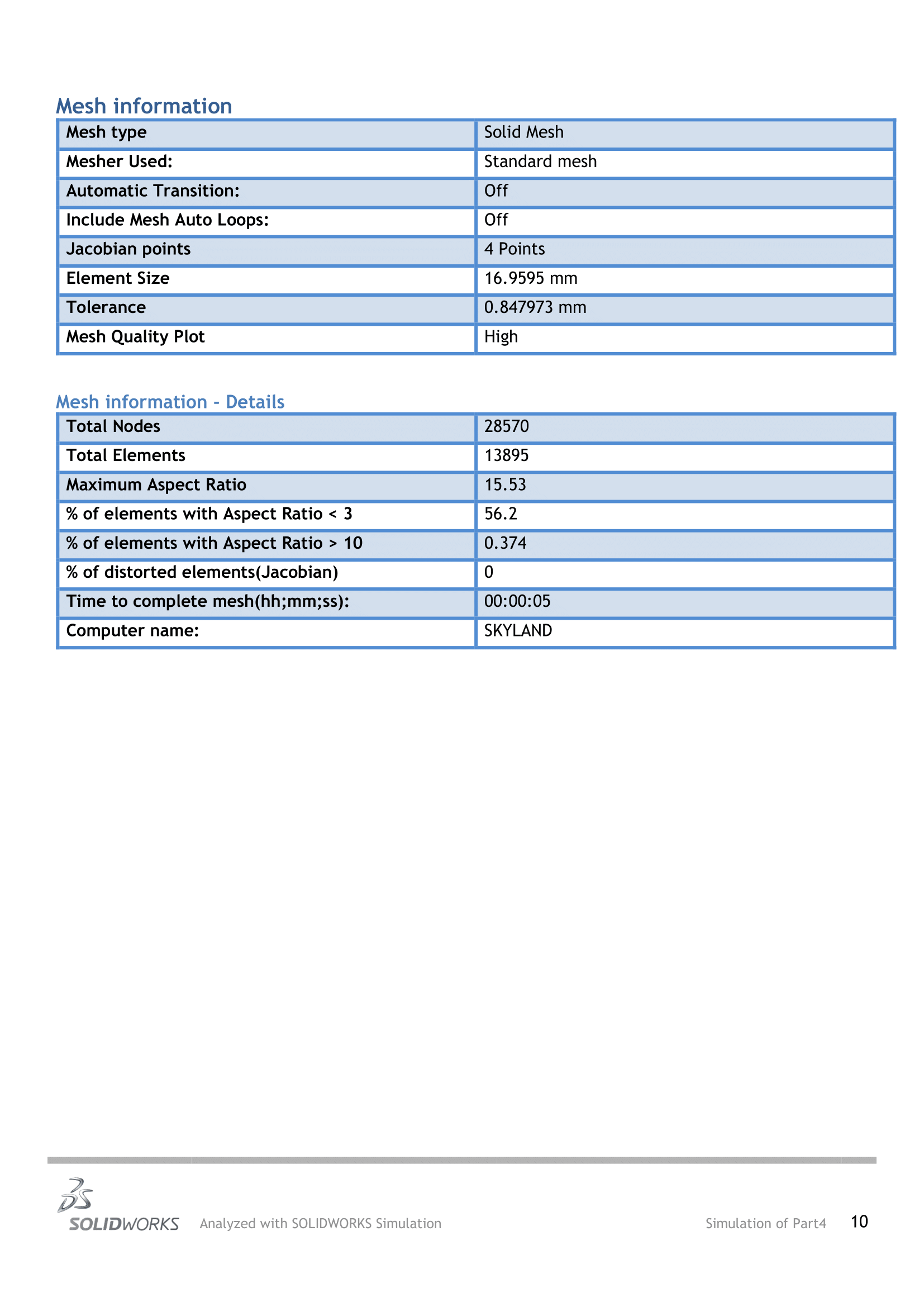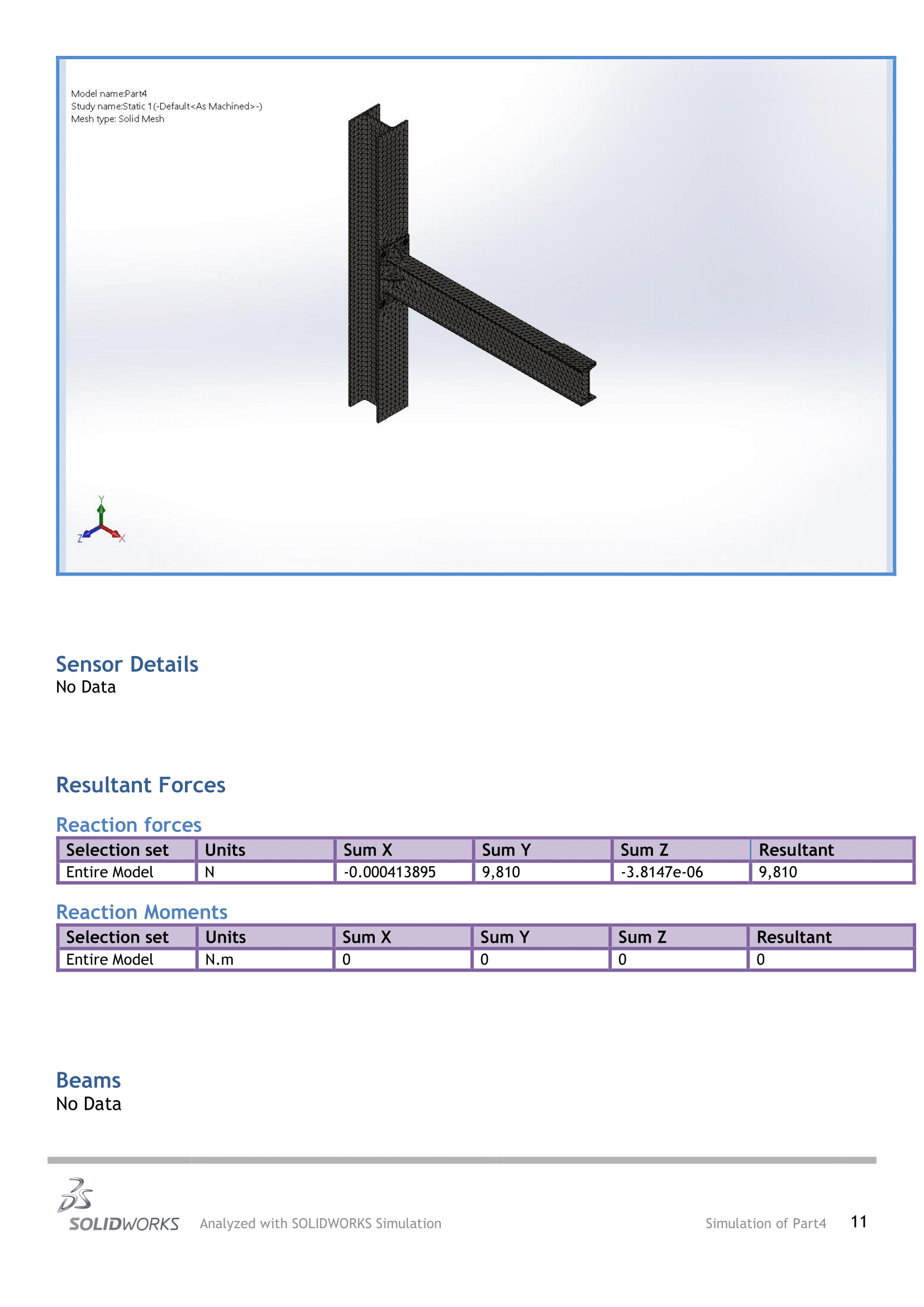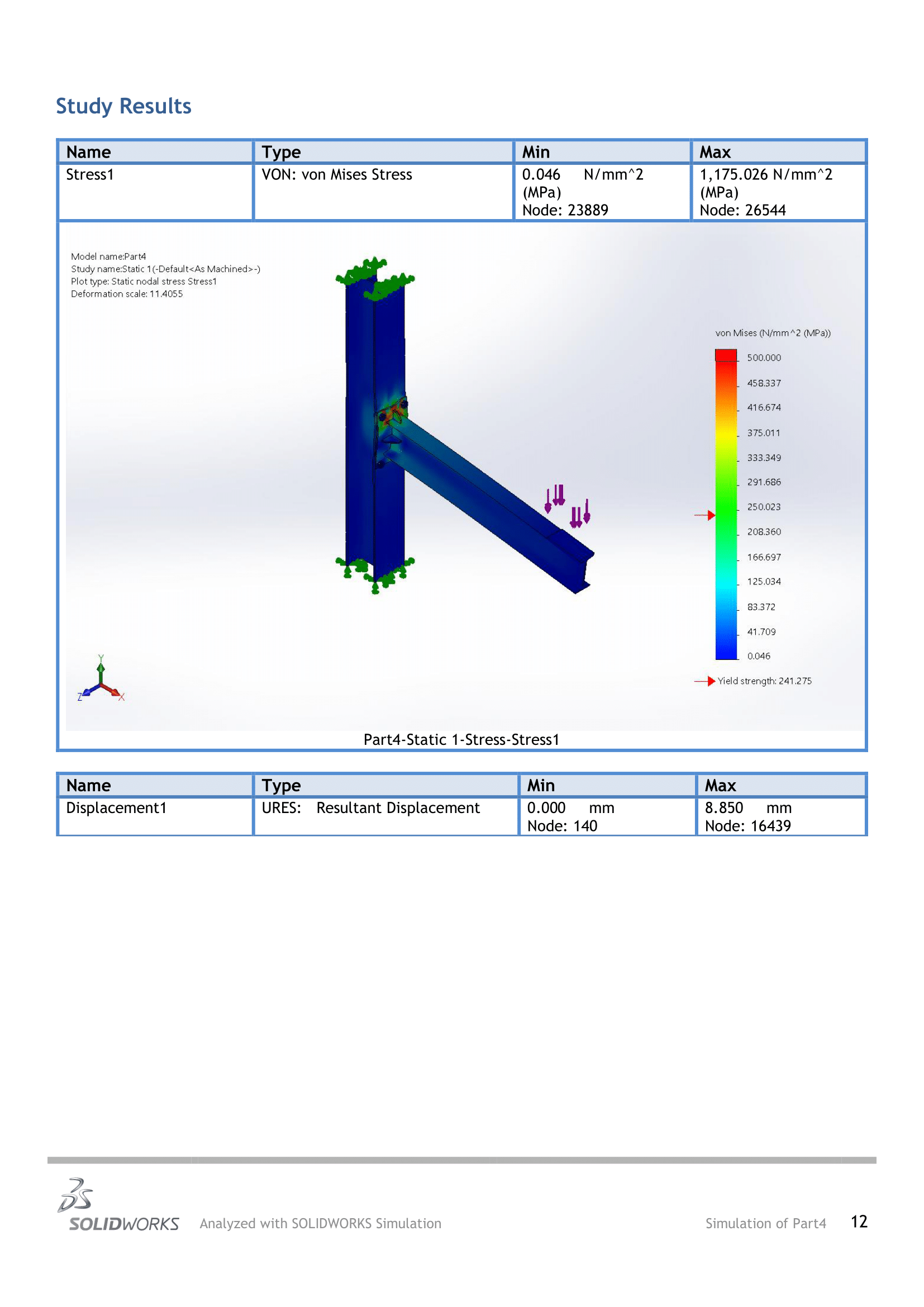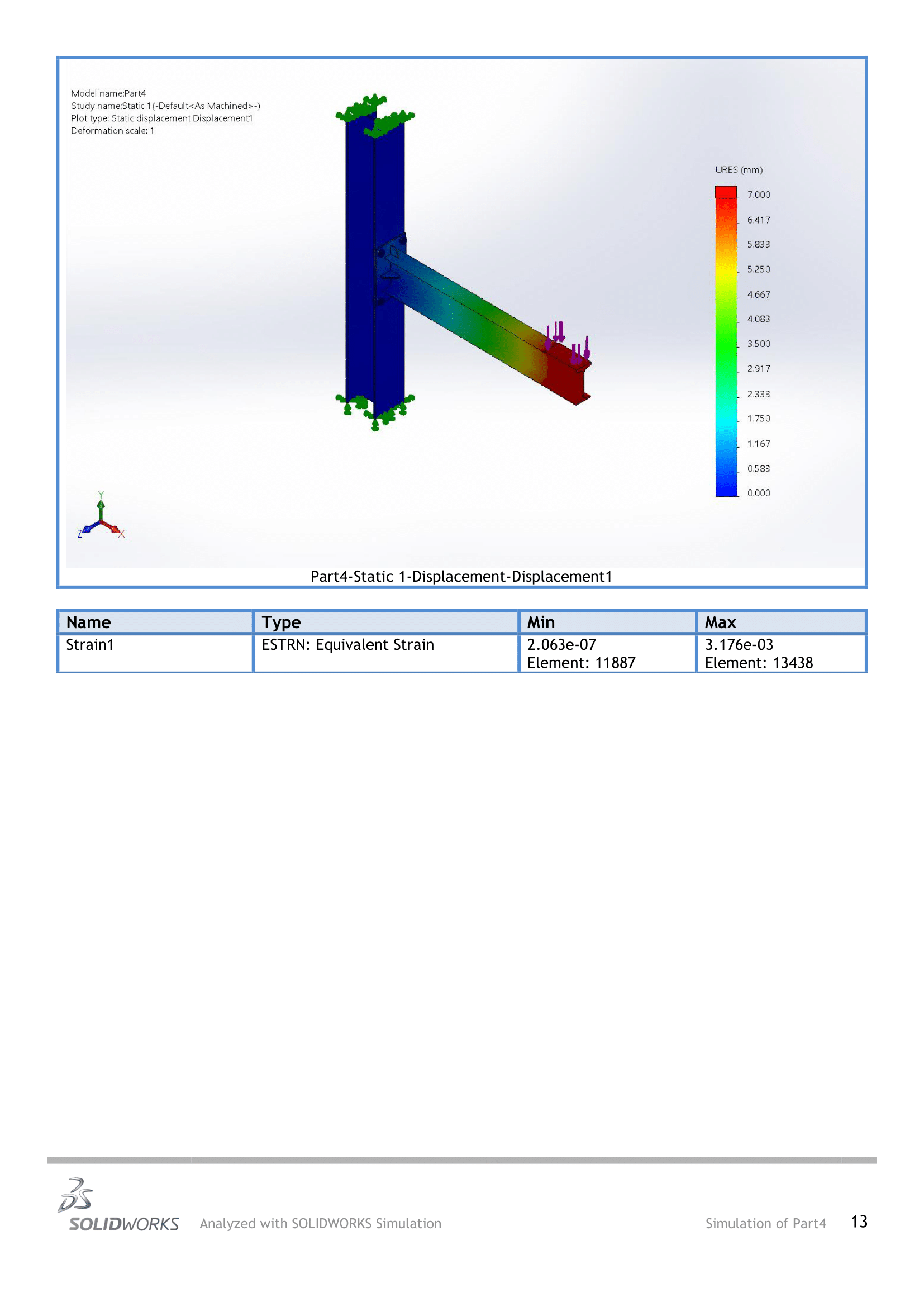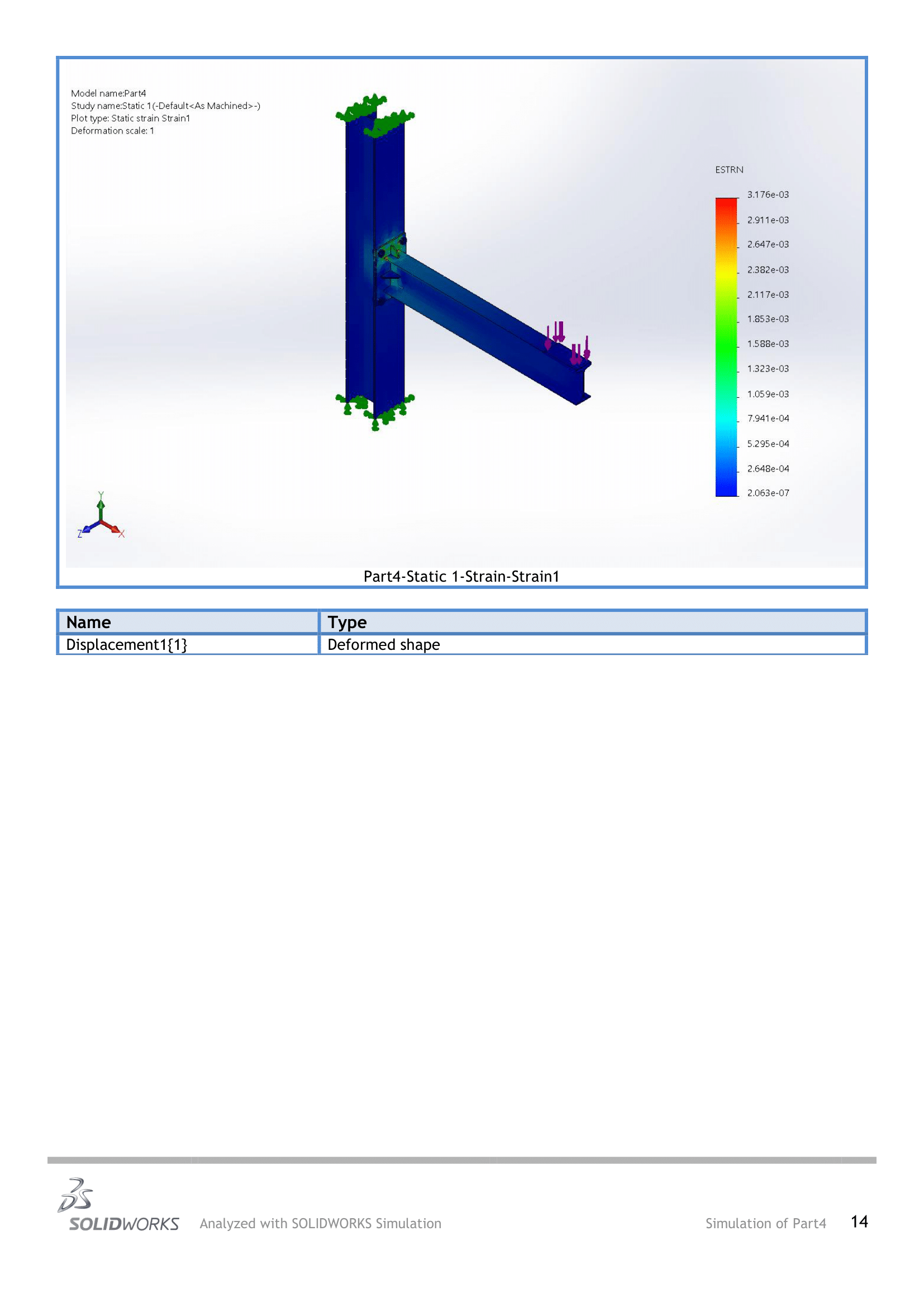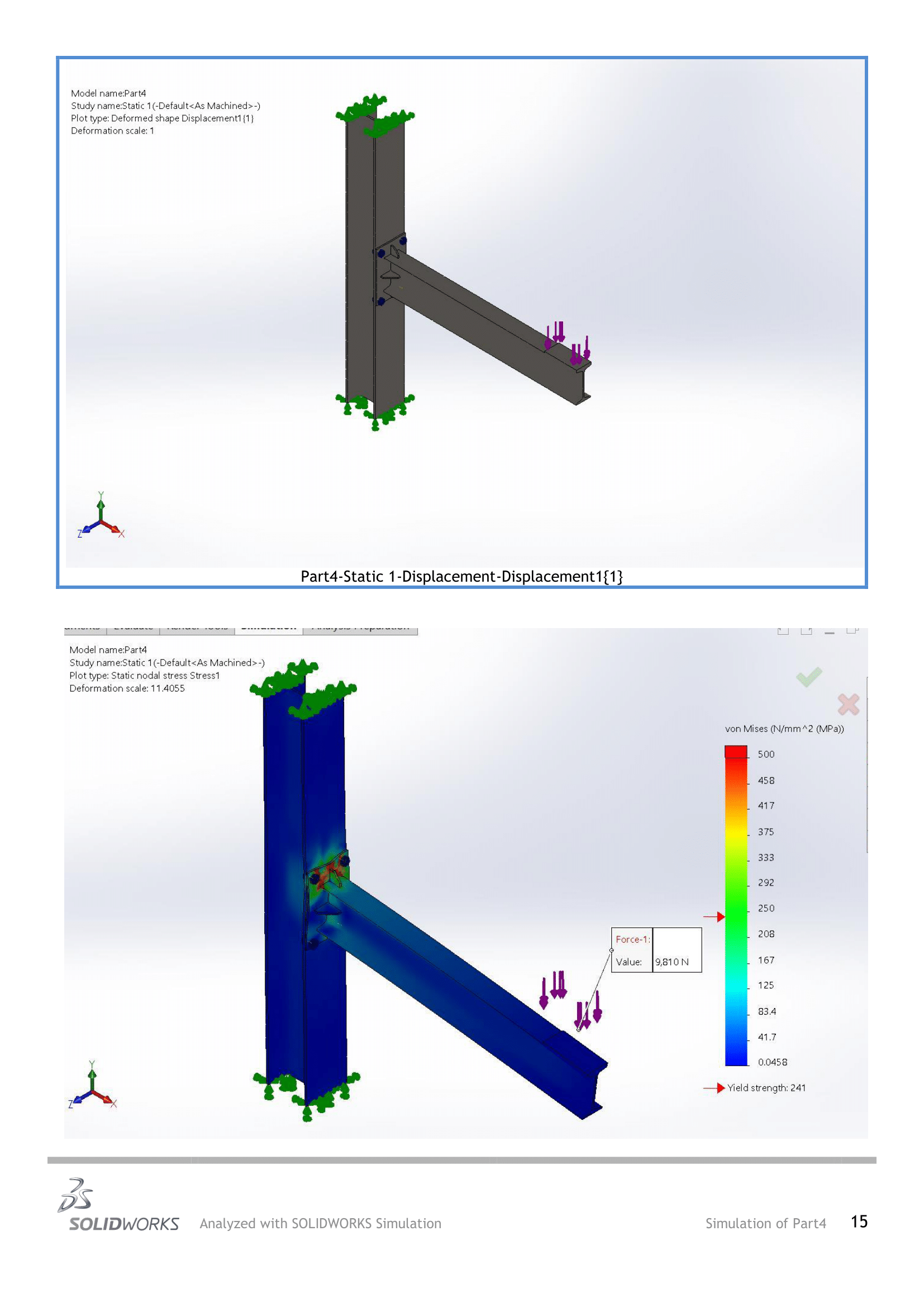# Simulation of I section beam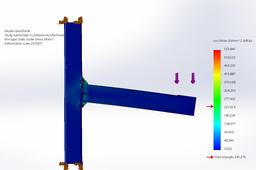Simulation and analysis of stress or failures in a beam.

1. ### Step 1:

Simulation steps

1. Create Model
2. Create Simulation Study
3. Define Boundry condition
5. Meshing
6. Run the Simulation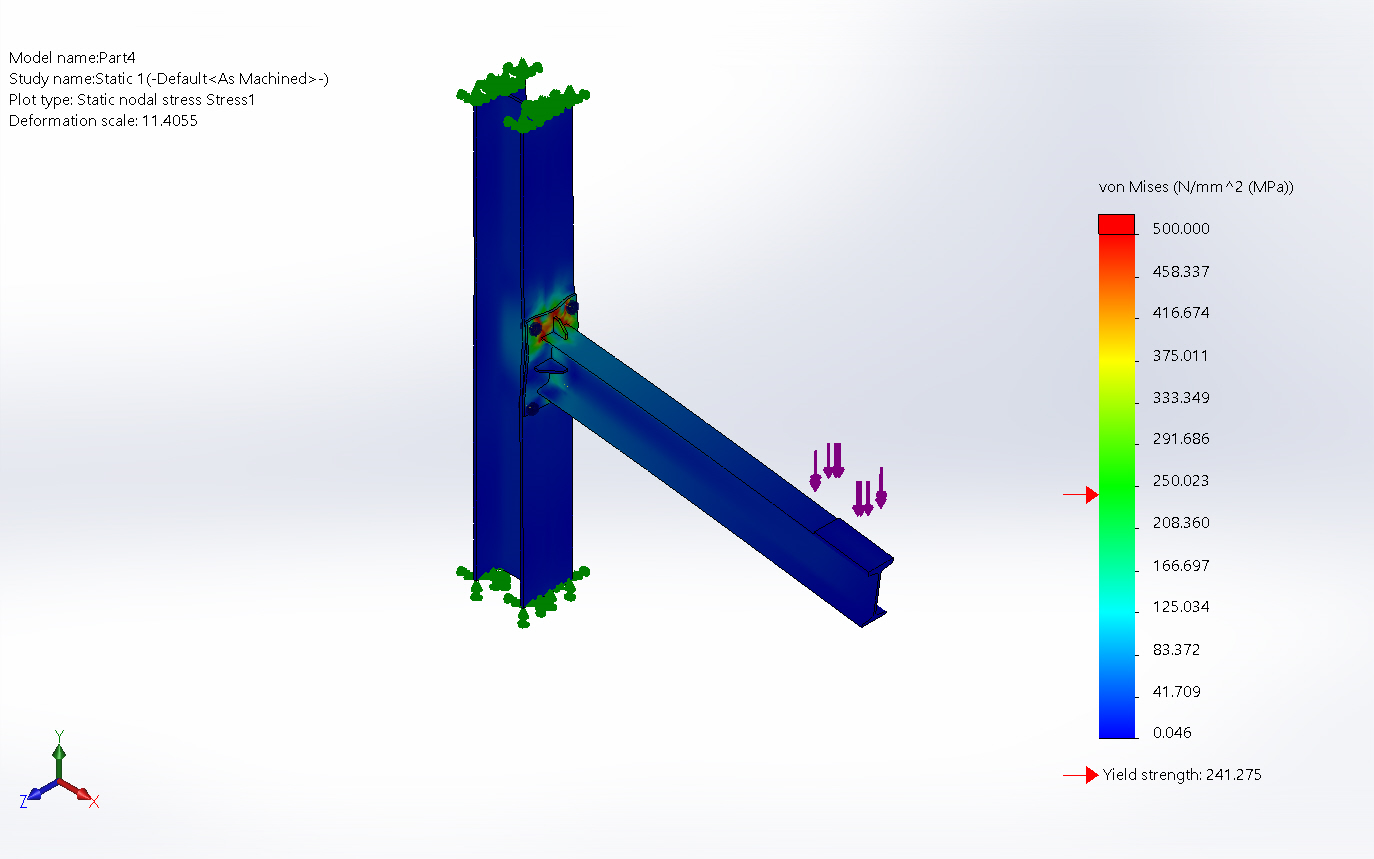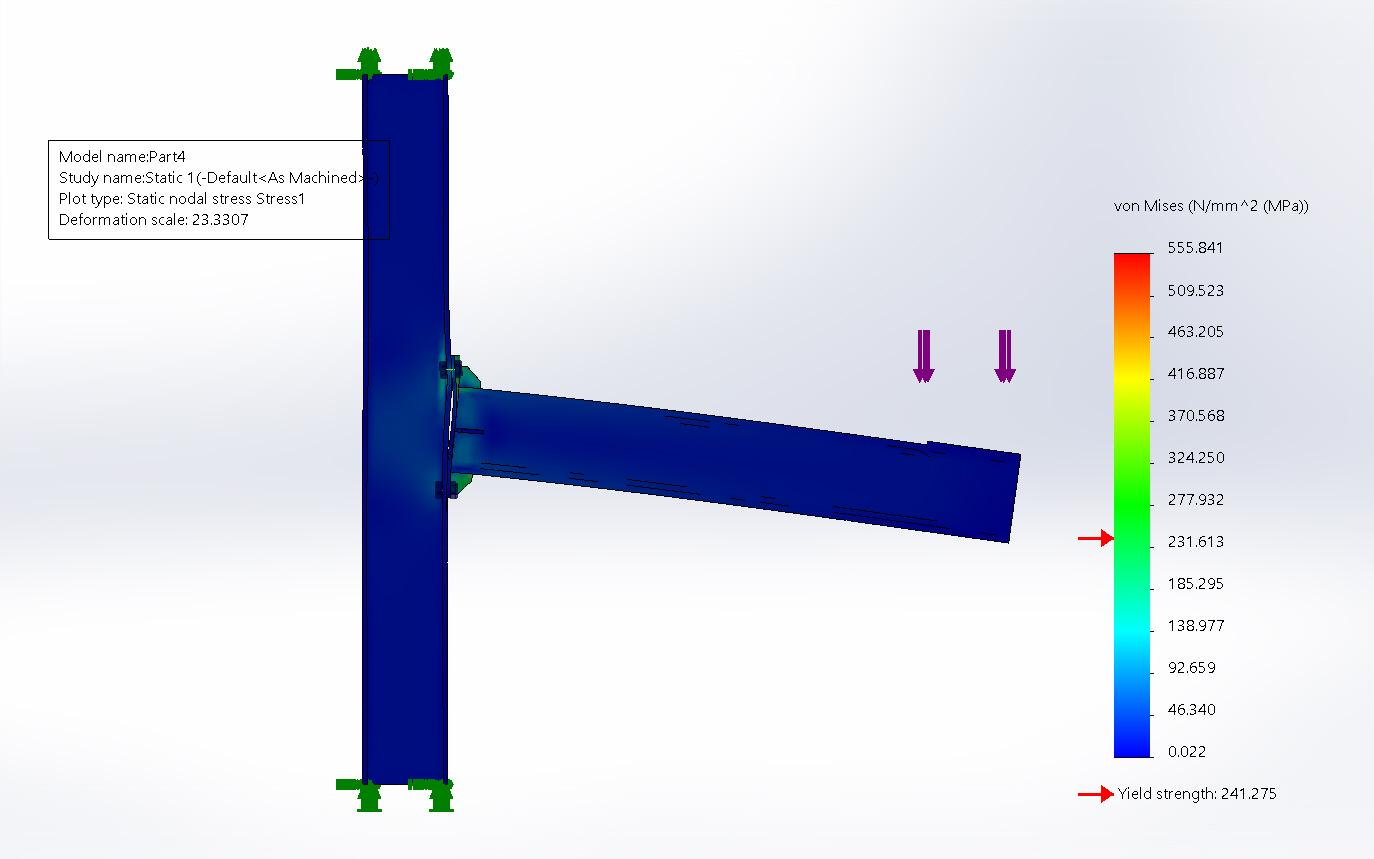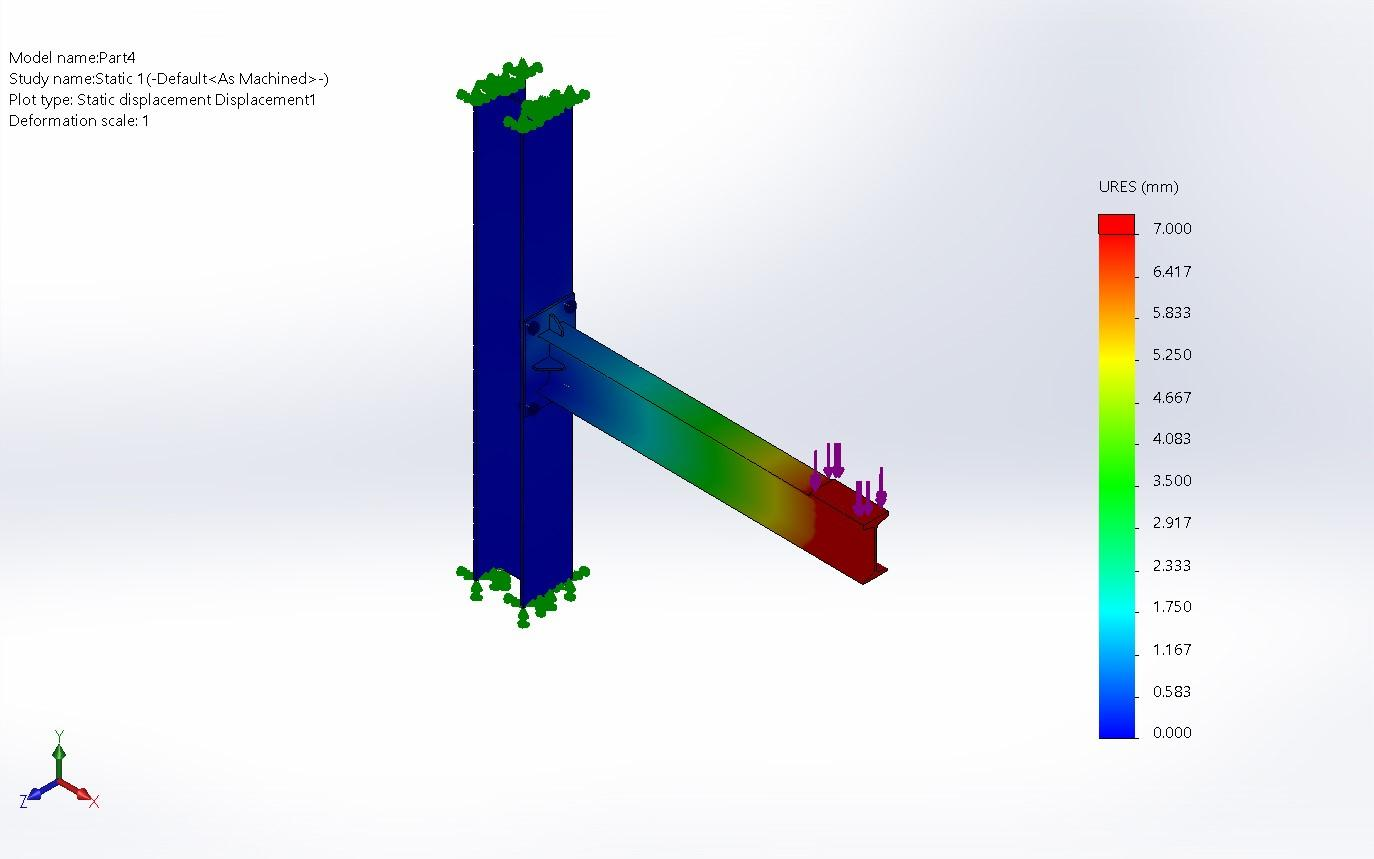Material: Cast Alloy Steel

Model type: Linear Elastic Isotropic

Resultant Forces: 9,810 N

Stress Analysis:

Type: VON- von Mises Stress

Max. stress: 1,175.026 N/mm^2 (MPa)

Max. Displacement: 8.850 mm

Yield strength: 2.41275e+08 N/m^2

Tensile strength: 4.48082e+08 N/m^2

Elastic modulus: 1.9e+11 N/m^2

Poisson's ratio: 0.26

Mass density: 7,300 kg/m^3

Shear modulus: 7.8e+10 N/m^2

Thermal expansion coefficient: 1.5e-05 /Kelvin

2. ### Step 2: End Results